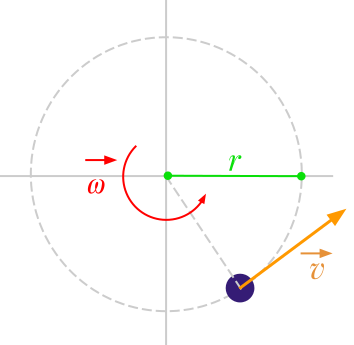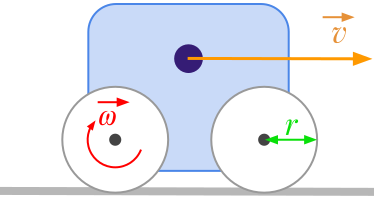Question #462

# How to convert meters per second to radians per second?

Merged questionsThe formula to convert linear speed ﻿﻿ expressed in meters per second [﻿﻿] to angular velocity ﻿﻿ expressed in radians per second [﻿﻿] is given by:

﻿﻿

where:

• ﻿﻿ is the linear velocity expressed in meters per second (﻿﻿)
• ﻿﻿ is the angular velocity expressed in radians per second (﻿﻿)
• ﻿﻿ is the radius expressed in meters (﻿﻿)For example, let's considere a vehicle with wheels having a radius of 10 centimetres. If the vehicle is moving at the linear velocity of 2 m/s, the rotation speed of the wheels is given by:

﻿﻿

14 events in history
Answer by Alphonsio 12/14/2020 at 07:14:55 PMThe formula to convert linear speed ﻿﻿ expressed in meters per second [﻿﻿] to angular velocity ﻿﻿ expressed in radians per second [﻿﻿] is given by:

﻿﻿

where:

• ﻿﻿ is the linear velocity expressed in meters per second (﻿﻿)
• ﻿﻿ is the angular velocity expressed in radians per second (﻿﻿)
• ﻿﻿ is the radius expressed in meters (﻿﻿)For example, let's considere a vehicle with wheels having a radius of 10 centimetres. If the vehicle is moving at the linear velocity of 2 m/s, the rotation speed of the wheels is given by:

﻿﻿

Answer by Alphonsio 12/14/2020 at 07:14:11 PMThe formula to convert linear speed ﻿﻿ expressed in meters per second [﻿﻿] to angular velocity ﻿﻿ expressed in radians per second [﻿﻿] is given by:

﻿﻿

where:

• ﻿﻿ is the linear velocity expressed in meters per second (﻿﻿)
• ﻿﻿ is the angular velocity expressed in radians per second (﻿﻿)
• ﻿﻿ is the radius expressed in meters (﻿﻿)For example, let's considere a vehicle with wheels having a radius of 10 centimetres. If the vehicle is moving at the linear velocity of 2 m/s, the rotation speed of the wheels is given by:

﻿﻿

Answer by Alphonsio 12/14/2020 at 07:10:43 PMThe formula to convert linear speed ﻿﻿ expressed in meters per second [﻿﻿] to angular velocity ﻿﻿ expressed in radians per second [﻿﻿] is given by:

﻿﻿

where:

• ﻿﻿ is the linear velocity expressed in meters per second (﻿﻿)
• ﻿﻿ is the angular velocity expressed in radians per second (﻿﻿)
• ﻿﻿ is the radius expressed in meters (﻿﻿)For example, if a vehicle with wheels having a radius of 10 centimertres is moving at 2 m/s, the rotation speed of the wheels is given by:

﻿﻿

Answer by Alphonsio 12/14/2020 at 07:10:27 PMThe formula to convert linear speed ﻿﻿ expressed in meters per second [﻿﻿] to angular velocity ﻿﻿ expressed in radians per second [﻿﻿] is given by:

﻿﻿

where:

• ﻿﻿ is the linear velocity expressed in meters per second (﻿﻿)
• ﻿﻿ is the angular velocity expressed in radians per second (﻿﻿)
• ﻿﻿ is the radius expressed in meters (﻿﻿)﻿

For example, if a vehicle with wheels having a radius of 10 centimertres is moving at 2 m / s, the rotation speed of the wheels is given by:

﻿﻿

Answer by Alphonsio 12/14/2020 at 07:10:22 PM﻿

The formula to convert linear speed ﻿﻿ expressed in meters per second [﻿﻿] to angular velocity ﻿﻿ expressed in radians per second [﻿﻿] is given by:

﻿﻿

where:

• ﻿﻿ is the linear velocity expressed in meters per second (﻿﻿)
• ﻿﻿ is the angular velocity expressed in radians per second (﻿﻿)
• ﻿﻿ is the radius expressed in meters (﻿﻿)For example, if a vehicle with wheels having a radius of 10 centimertres is moving at 2 m / s, the rotation speed of the wheels is given by:

﻿﻿

Answer by Alphonsio 12/12/2020 at 05:41:38 AM

The formula to convert linear speed ﻿﻿ expressed in meters per second [﻿﻿] to angular velocity ﻿﻿ expressed in radians per second [﻿﻿] is given by:

﻿﻿

where:

• ﻿﻿ is the linear velocity expressed in meters per second (﻿﻿)
• ﻿﻿ is the angular velocity expressed in radians per second (﻿﻿)
• ﻿﻿ is the radius expressed in meters (﻿﻿)

Answer by Alphonsio 12/12/2020 at 05:40:57 AM

The formula to convert linear speed ﻿﻿ expressed in meters per second [﻿﻿] to angular velocity ﻿﻿ expressed in radians per second [﻿﻿] is given by:

﻿﻿

where:

• ﻿﻿ is the linear velocity expressed in meters per second (﻿﻿)
• ﻿﻿ is the angular velocity expressed in radians per second (﻿﻿)
• ﻿﻿ is the radius expressed in meters (﻿﻿)

Answer by Alphonsio 12/12/2020 at 05:39:53 AM

The formula to convert linear speed ﻿﻿ expressed in meters per second [﻿﻿] to angular velocity ﻿﻿ expressed in radians per second [﻿﻿] is given by:

﻿﻿

where:

• ﻿﻿ is the linear velocity expressed in meters per second (﻿﻿)
• ﻿﻿ is the angular velocity expressed in radians per second (﻿﻿)
• ﻿﻿ is the radius expressed in meters (﻿﻿)

Answer by Alphonsio 12/11/2020 at 07:45:42 PM

The formula to convert linear speed expressed in meters per second [﻿﻿] to angular velocity expressed in radians per second [﻿﻿] is given by:

﻿﻿

﻿﻿

where:

• ﻿﻿ is the linear velocity expressed in meters per second (﻿﻿)
• ﻿﻿ is the angular velocity expressed in radians per second (﻿﻿)
• ﻿﻿ is the radius expressed in meters (﻿﻿)

Answer by Alphonsio 12/11/2020 at 07:41:46 PM

The formula to convert linear speed expressed in meters per second [﻿﻿] to angular velocity expressed in radians per second [﻿﻿] is given by:

﻿﻿

﻿﻿

where:

• ﻿﻿ is the linear velocity expressed in meters per second (﻿﻿)
• ﻿﻿ is the angular velocity expressed in radians per second (﻿﻿)
• ﻿﻿ is the radius expressed in meters (﻿﻿)

Answer by Alphonsio 12/11/2020 at 07:39:52 PM

The formula to convert linear speed expressed in meters per second [﻿﻿] to angular velocity expressed in radians per seconde [﻿﻿] is given by:

﻿﻿

﻿﻿

where:

• ﻿﻿ is the linear velocity expressed in meters per second (﻿﻿)
• ﻿﻿ is the angular velocity expressed in radians per second (﻿﻿)
• ﻿﻿ is the radius expressed in meters (﻿﻿)

Question by Alphonsio 12/11/2020 at 07:39:09 PM
How to convert m/s to rad/s?
Answer by Alphonsio 12/11/2020 at 07:38:51 PM

The formula to convert linear speed expressed in meters per second [﻿﻿] to angular velocity expressed in radians per seconde [﻿﻿] is given by:

﻿﻿

﻿﻿

where:

• ﻿﻿ is the linear velocity expressed in meters per second (﻿﻿)
• ﻿﻿ is the angular velocity expressed in radians per second (﻿﻿)
• ﻿﻿ is the radius expressed in meters (﻿﻿)

Question by Alphonsio 12/11/2020 at 07:34:38 PM
How to convert meters per second to radians per second?

Icons proudly provided by Friconix.